# Reference Design for Model Based PID Tuning

Publish Date: Apr 03, 2015 | 3 Ratings | 5.00 out of 5 | Print | Submit your review

## Overview

Nearly all control systems for industrial or embedded applications centers on the use of some form of proportional integral derivative (PID) control. The ubiquitous control algorithm can be applied to nearly any system type and when tuned properly will provide stable control over a large range of the system workspace. The design presented here along with the case study will provide a starting point for advanced analytical methods that ensure the controller functions as expected.

### 1. Introduction

Finding the proper gains for a PID control system can often prove difficult.  Often it takes an experienced operator, or lengthy tuning process and a bit of luck to achieve a well-tuned system that balances speed of response with stability while minimizing steady-state error.  In addition to that, the system might require different gains for different operating regions.  To simplify this process, the following will present an analytical approach to generate a system model and set the PID gains based on desired system performance characteristics.  This process can be repeated frequently or even in real-time to create a control system that continually adjusts the PID gains to maintain a desired system performance.  The real time modeling can also be used to check for fault conditions as the system changes over time,.

### 2. High-Level PID Background

The following diagram shows a basic closed loop controller.  An input setpoint is given; the error (difference between the setpoint and the output of the system) is found and used to calculate a control effort that is then applied to the plant.  The output of the system is fed back to calculate the error.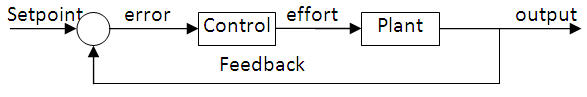PID control uses the error to calculate three components of the output that correspond to the proportional, integral and derivative feedback, components that give the control scheme its name.  The equation for PID control often takes the following form in the time domain.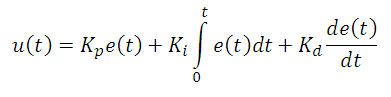The first component behaves like a spring, generating a control output that acts to force the system to the setpoint.  The second component integrates the error from the starting time to the current time and supplies control effort to drive steady state error to zero.    The final component generates control output proportional to change in the error, essentially behaving like an artificial damper.  The key is to adjust the three gains (Kp, Ki and Kd) to weight the three components and to achieve the desired performance characteristics while avoiding system instability.

PID tutorial

PID Explanation

### 3. System Modeling

The first step in a model-based approach to tuning a PID controller begins with the definition of a system model.  The most robust version of system identification uses the tools built into the Control Design and Simulation Toolkit.  This method produces valid results over a broad range of system types and stimuli, but it can involve a heavy processing load.  The approach presented here focuses on an open loop step response of a first order system, the identification of which can be done with a simple exponential fitting function, an implementation of which is included here.

The first order model with time delay has a transfer function in the continuous domain with following form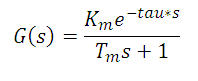Where Km is the system gain, tau is the time delay and Tm is the system time constant.  In the time domain, the first order response to a step function (with delay) translates to the following form, defined specifically in terms that are geared for a DC motor system: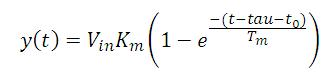Where Vin is the size of the input step, t is the system time, and t0 is the time of the step.  The following graph represents a typical first order step response of a DC motor taking voltage as an input and providing a tachometer value for feedback.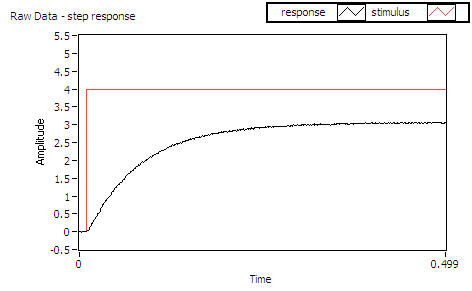In this example, the time delay (tau) is very small, and the system reaches a stable steady-state value; however these aren’t requirements for the simplified system identification algorithm.  The following image shows the block diagram of “curve fitting.vi.”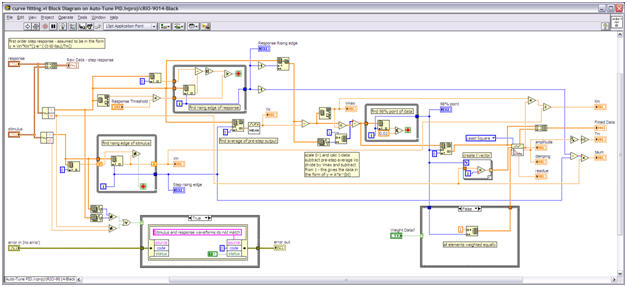The value of tau can be calculated by finding the time between the rising edge of stimulus step and the rising edge of the response.  The response rising edge is defined as a percentage of the final steady-state value set by the value of “Response Threshold”, and the algorithm uses the maximum value of the response, Vmax, as an approximation of the steady-state value.  “Response Threshold” has a default value of 2% and can be changed as an optional input if the system has a high amount of noise.

The average steady-state value of the response before the step input (Vo) is used along with Vmax to scale the step response to values.  The system is considered to have settled when it reaches 98% of Vo.  The algorithm performs three manipulations to put the post-step response data Yraw, subtracting the pre-step steady state value, dividing by Vmax and subtracting from 1.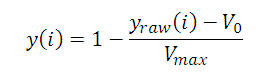This guarantees that the data be in the form of an exponential decay from 1 to 0 that will be fit to the following equation.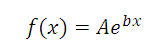When the manipulated response data fits to the exponential equation, the following relationships yield the model parameters, and the residue, an optional output of this system identification block, gives a quality of the fit provided by this method.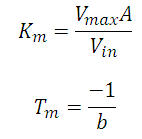For systems that don’t perfectly match the ideal first order model, the optional weighting vector can be used to focus the fit on first and last 20% of the step response.  This option in turn focuses the fitting on the fast dynamic behavior and the steady-state behavior of the system.

### 4. Calculating PID Gains

A brief literature search will uncover hundreds of methods for scheduling PID gains based on modeling, the first and most well-known of which is the Zeigler and Nichols approach.  The code in the following case study also contains other more complex methods for PID gain calculation, the references for which are included in the code comments.  The specific tuning methods used in the case study provide equations for the PID gains based on a first order model of the system with delay and an academic version of the PID controller in the following form.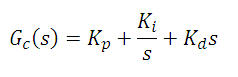### 5. Ensure Stability in Deployed Controller

For higher risk applications the final task before deploying the controller should be to simulate the entire open and closed loop systems, comparing the models to actual data and experimenting with gain scheduling algorithms.  The discussion thus far has focused on the time domain and the s-domain; however most modern control systems (and therefore the implementation of most modern controllers) exist in the digital or discrete domain.  Furthermore, the implementation of many PID controllers doesn’t perfectly match the simple academic representation above.  Frequently a set of theoretical PID gains found in the s-domain will transfer to the digital domain and produce acceptable results.  However for systems with very tight performance requirements or for controllers with very high loop rates such as those often implemented on FPGA, it is advisable to simulate the closed-loop response of the system.  A detailed discussion of the National Instruments simulation tools can be found at the following link:

http://zone.ni.com/devzone/cda/tut/p/id/6931

### 6. Case Study – Thermal System

The following description and the attached code will walk through the steps to implement a model-based PID tuning algorithm on a simple thermal system.  The following image shows the setup that consists of a heat source (a halogen lamp with its intensity controlled with by a PWM signal) and a thermocouple in a plexiglass box.  A small computer case fan provides a controllable airflow (also via PWM signal) through the system.  A CompactRIO provides the processing and IO for data acquisition in the system identification phase through the control deployment.  The design uses a cRIO-9014 coupled with a cRIO-9104 as the base of the system (this could easily be replaced by a different cRIO controller or a different backplane) with an NI-9212 thermocouple module and an NI-9472 DIO module.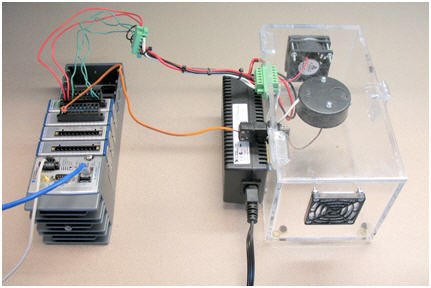The file “RT thermal sys ID and autotune.vi” automates the entire process of generation of a step response, calculation of a 1st order model based on that step response, determination of PID gains based on the model and PID control of that system based on the calculated gains.  After running the VI, the user needs to wait for the system to reach a steady state before clicking the “steady state?” button.  After that, the automated process yields a controlled system.

##### Step 1 – Create an open-loop system and collect the stimulus / response data.

The included file “FPGA IO – thermal.vi” acts as an FPGA driver for the system, generating PWM outputs to control the fan and the light as well as reading the raw data from thermocouple module.  The raw data gets passed to the Real-Time controller of the cRIO where the calibration and cold-junction compensation values are used to translate the raw data into temperature values in degrees Celsius.  The following screen shot shows the block diagram of the FPGA code.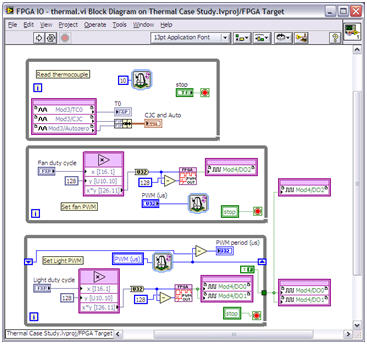##### Step 2 – Fit the stimulus / response data to the system model.

This example uses two methods to fit the data to a first order system model. The first method uses the system identification tools built into Control Design and Simulation Toolkit, and the second method uses the simple curve fit algorithm discussed earlier.  The native system identification tools provide a much more robust solution that is valid over a large range of stimulus / response data; however for a step response of a 1st order system, the algorithm can be simplified significantly to produce an approximate model using far fewer calculations.  The function “curve fitting.vi” does this simple system identification and generates a first order model with a time delay.  The simulation contained in “simulate OL – plants.vi” compares a collected data to a model calculated using the Control Design and Simulation tools and to a model calculated using the simple curve fitting approach.  This VI also contains data from a DC motor system to illustrate that the approach works for systems of different speeds.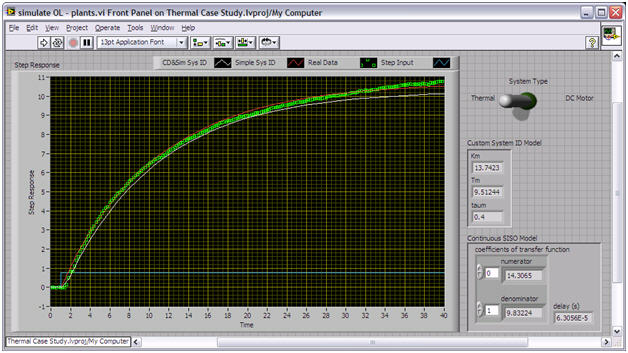##### Step 3 – Empirically define the PID gains using the approximated model.

With an approximation of the model, the PID gains can then be calculated using many different methods.  The function “PID tuning algorithms.vi” contains five different algorithms, each of which might produce the best results for a specific type of system.

##### Step 4 (optional) – Simulate the closed-loop response with various PID gains and tuning methods.

Completing an offline simulation of the closed loop system allows the designer to explore which PID tuning algorithm might be the most effective for the specific system.  The function “simulate CL – thermal system.vi” compares the continuous and discrete closed loop simulations of a plant and controller to the closed loop system using the actual FPGA PID block.  The academic representation of a PID controller (both in the discrete and in the continuous domains) differs slightly from the FPGA implementation.  Simulating these implementations together assists the designer in understand and tuning the system.  The screen shot shown in ‘Step 6’ includes the simulated open loop system as well as collected data.

##### Step 5 – Implement the PID gains to create a real control system.

In the function “RT thermal sys ID and autotune.vi” the first half of the code uses the FPGA as an IO tool.  However a new FPGA bitfile containing the PID control algorithm is loaded in the second part.  The function “FPGA PID – thermal.vi” uses the same basic code as the other FPGA VI, but it includes a PID control loop that receives the setpoint and process variable information from the Real-Time controller code.

##### Step 6 (optional) – Compare the physical results to the simulated system.

The function “Simulate CL – thermal system.vi” contains data showing the fit of the simulated system to the actual system.  The yellow line in each of the graphs represents real collected data and provides an offline check to validate the accuracy of the model and the tuning method.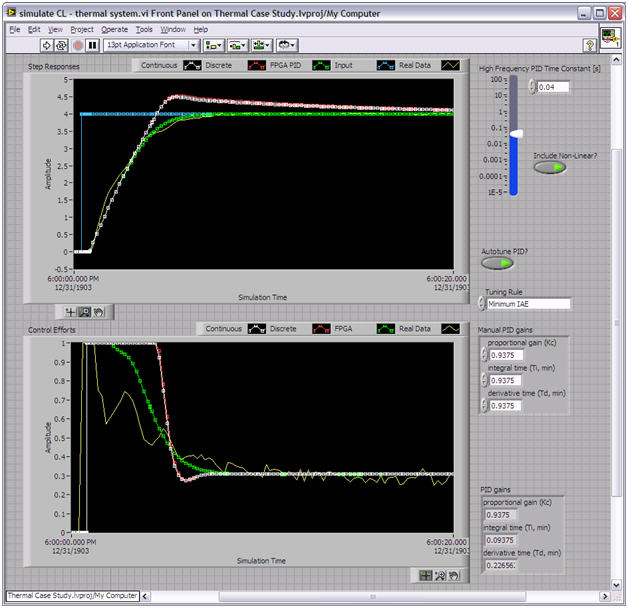### 7. Conclusions

The effectiveness of the approach presented in this document has a high dependence on the quality of the model and on the tuning algorithm chosen.  Simple tools like the ones shown in the case study work well on a well-behaved first order system, but the approach can be scaled to work on more complex systems.  Using the LabVIEW system identification tools and the Control Design and Simulation Toolkit, models can be generated for higher order systems using more generic input waveforms.  The most complex level of system identification could be done in real time using the recursive functions in the toolkit.  Similarly, increasing complexity of the analytical methods for PID gain scheduling could improve performance over a wider range of system types.  Regardless, this case study provides a starting point for implementation of model-based PID tuning.

### 8. Feedback

This reference application was created by the NI Systems Engineering group.

Please submit your feedback in the Model Based PID Tuning Forum so that we can improve this component for future applications.

Please direct support questions to NI Technical Support.